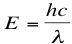# 2. Photon Energy and Flux

$$\newcommand{\vecs}{\overset { \rightharpoonup} {\mathbf{#1}} }$$ $$\newcommand{\vecd}{\overset{-\!-\!\rightharpoonup}{\vphantom{a}\smash {#1}}}$$$$\newcommand{\id}{\mathrm{id}}$$ $$\newcommand{\Span}{\mathrm{span}}$$ $$\newcommand{\kernel}{\mathrm{null}\,}$$ $$\newcommand{\range}{\mathrm{range}\,}$$ $$\newcommand{\RealPart}{\mathrm{Re}}$$ $$\newcommand{\ImaginaryPart}{\mathrm{Im}}$$ $$\newcommand{\Argument}{\mathrm{Arg}}$$ $$\newcommand{\norm}{\| #1 \|}$$ $$\newcommand{\inner}{\langle #1, #2 \rangle}$$ $$\newcommand{\Span}{\mathrm{span}}$$ $$\newcommand{\id}{\mathrm{id}}$$ $$\newcommand{\Span}{\mathrm{span}}$$ $$\newcommand{\kernel}{\mathrm{null}\,}$$ $$\newcommand{\range}{\mathrm{range}\,}$$ $$\newcommand{\RealPart}{\mathrm{Re}}$$ $$\newcommand{\ImaginaryPart}{\mathrm{Im}}$$ $$\newcommand{\Argument}{\mathrm{Arg}}$$ $$\newcommand{\norm}{\| #1 \|}$$ $$\newcommand{\inner}{\langle #1, #2 \rangle}$$ $$\newcommand{\Span}{\mathrm{span}}$$

Light, which we know travels at speed c in a vacuum, has a frequency f and a wavelength λ. Frequency can be related to the wavelength by the speed of light in the equationThe energy of a photon, as described in The Basics of Quantum Theory, is given by the equationorThis means just what we said before in “The Electromagnetic Spectrum”, that the higher the frequency and shorter the wavelength, the greater the energy of the photon of light. Knowing the relation between the energy and frequency/wavelength of light is important when it comes to figuring out the spectrum of light and energy emitted by the sun. It is worth noting that the energy of a photon is often measured in electron volts (eV), which is the amount of energy needed to move an electron across 1 Volt.

Photon flux is another important concept because it allows us to understand how much light actually comes into contact with our devices on the surface of the earth, and therefore how many electrons can be generated for current flow. Flux is a fancy word for the amount of something coming into contact with or passing through a given surface area; therefore the photon flux is just the amount of photons hitting the surface of a solar cell in a given time. The photon flux is given by the equation:where Φ is the photon flux, which is defined as the number of photons per second per square meter.The above picture illustrates the idea of the inverse square law, by showing how the flux of these lines through a given area decreases with distance from the source. This gives a good representation for how flux can be pictured, and shows how flux can give an indication of the intensity of light on a surface. In our case, intensity would decrease as light travels from the sun, which is an application of flux that we will discuss in later sections.

Source: <en.Wikipedia.org/wiki/File:In...square_law.svg>

2. Photon Energy and Flux is shared under a not declared license and was authored, remixed, and/or curated by LibreTexts.